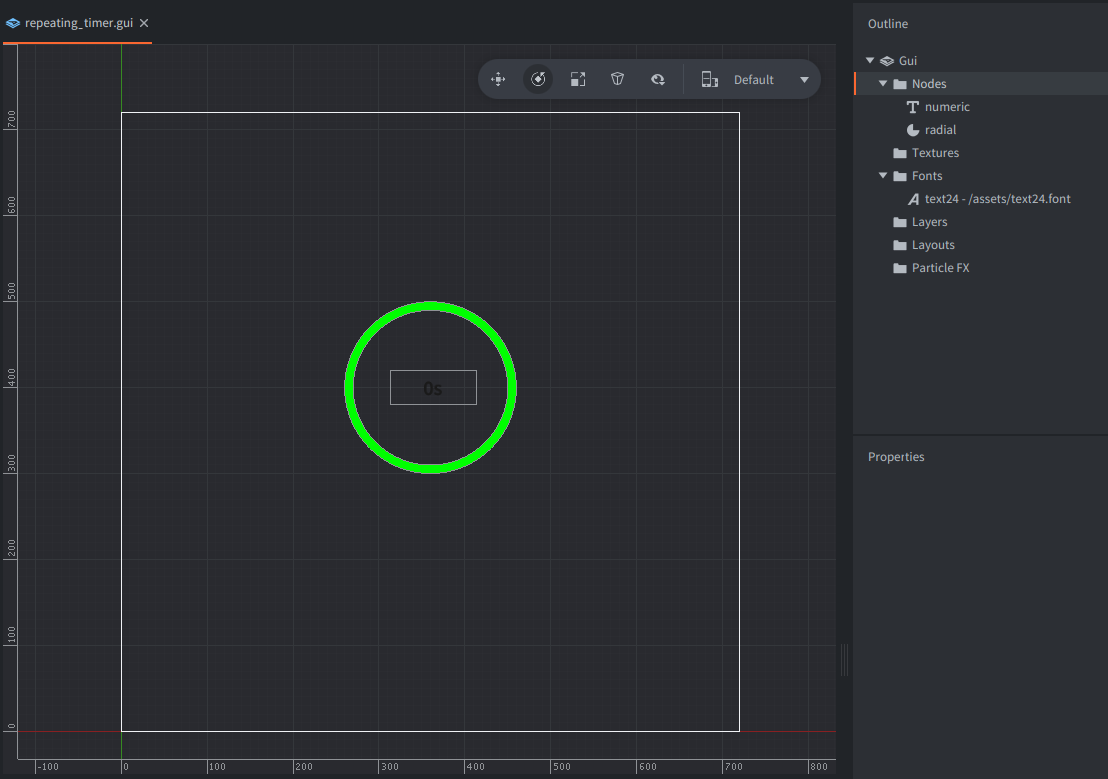#### Setup

The example shows how to use Defold built-in timer and uses two indicators:

1. A numerical time text with seconds counter created using a text node
2. A circular time indicator created using a pie node

The timer trigers updates of those two indicators every 1s to form a counter.#### Scripts

repeating_timer.gui_script

```-- set value of numeric time indicator (from 0 to 60s)
local function update_numeric(p)
local node = gui.get_node("numeric")
gui.set_text(node, tostring(p) .. "s")
end

-- update radial/circle time indicator by changing the fill angle
local angle = p * 6
gui.set_fill_angle(node, angle)
end

function init(self)
self.count = 0			-- <1>
local interval = 1		-- <2>
local repeating = true	-- <3>

timer.delay(interval, repeating, function()		-- <4>
self.count = self.count + 1					-- <5>
local p = self.count % 60					-- <6>
update_numeric(p)							-- <7>
end)
end

--[[
1. Start the count with value 0.
2. We will use interval of 1 [s].
3. We will be repeating the timer endlessly.
4. Start the timer with interval (1s) and repeating (true) and pass a callback function.
5. The function will be called every 1s, so increase the count by 1 each time.
6. Get the modulo of 60, because the timer will be reset every 60s.
7. Update the numeric display of seconds passed.
8. Update the radial indicator of seconds passed.
--]]```

If you want to play with these examples, you can get the project on Github.

Do you want to see more examples? Why not write a few yourself and submit a pull request? We love contributions.